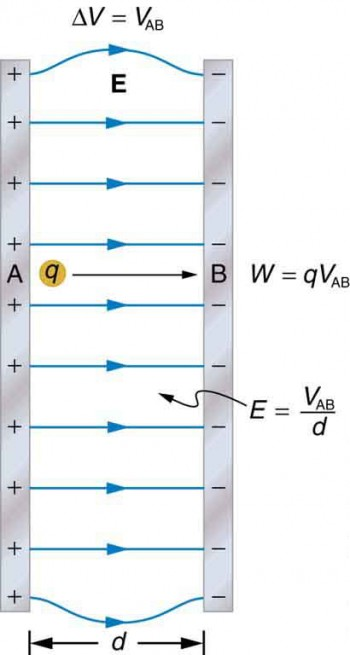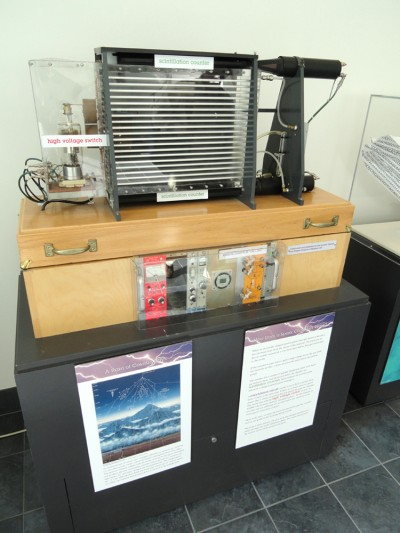Electric Potential in a Uniform Electric Field

Learning Objectives

By the end of this section, you will be able to:

• Describe the relationship between voltage and electric field.
• Derive an expression for the electric potential and electric field.
• Calculate electric field strength given distance and voltage.Figure 1. The relationship between V and E for parallel conducting plates is $E=\frac{V}{d}\\$. (Note that ΔV = VAB in magnitude. For a charge that is moved from plate A at higher potential to plate B at lower potential, a minus sign needs to be included as follows: –ΔV = VAVB = VAB. See the text for details.)

In the previous section, we explored the relationship between voltage and energy. In this section, we will explore the relationship between voltage and electric field. For example, a uniform electric field E is produced by placing a potential difference (or voltage) ΔV across two parallel metal plates, labeled A and B. (See Figure 1.)

Examining this will tell us what voltage is needed to produce a certain electric field strength; it will also reveal a more fundamental relationship between electric potential and electric field. From a physicist’s point of view, either ΔV or E can be used to describe any charge distribution. ΔV is most closely tied to energy, whereas E is most closely related to force. ΔV is a scalar quantity and has no direction, while E is a vector quantity, having both magnitude and direction. (Note that the magnitude of the electric field strength, a scalar quantity, is represented by E below.) The relationship between ΔV and E is revealed by calculating the work done by the force in moving a charge from point A to point B.

But, as noted in Electric Potential Energy: Potential Difference, this is complex for arbitrary charge distributions, requiring calculus. We therefore look at a uniform electric field as an interesting special case.

The work done by the electric field in Figure 1 to move a positive charge q from A, the positive plate, higher potential, to B, the negative plate, lower potential, is

W = −ΔPE = −qΔV.

The potential difference between points A and B is

−ΔV = −(VB − VA) = VA − VBVAB.

Entering this into the expression for work yields WqVAB.

Work is WFd cos θ; here cos θ = 1, since the path is parallel to the field, and so = Fd. Since = qE, we see that = qEd. Substituting this expression for work into the previous equation gives qEd = qVAB.

The charge cancels, and so the voltage between points A and B is seen to be

$\begin{cases}V_{\text{AB}}&=&Ed\\E&=&\frac{V_{\text{AB}}}{d}\end{cases}\\$ (uniform − field only)

where d is the distance from A to B, or the distance between the plates in Figure 1. Note that the above equation implies the units for electric field are volts per meter. We already know the units for electric field are newtons per coulomb; thus the following relation among units is valid: 1 N/C = 1 V/m.

Voltage between Points A and B

$\begin{cases}V_{\text{AB}}&=&Ed\\E&=&\frac{V_{\text{AB}}}{d}\end{cases}\\$ (uniform − field only)

where d is the distance from A to B, or the distance between the plates.

Example 1. What Is the Highest Voltage Possible between Two Plates?

Dry air will support a maximum electric field strength of about 3.0 × 106 V/m. Above that value, the field creates enough ionization in the air to make the air a conductor. This allows a discharge or spark that reduces the field. What, then, is the maximum voltage between two parallel conducting plates separated by 2.5 cm of dry air?

Strategy

We are given the maximum electric field E between the plates and the distance d between them. The equation VAB = Ed can thus be used to calculate the maximum voltage.

Solution

The potential difference or voltage between the plates is

VAB = Ed.

Entering the given values for E and d gives

VAB = (3.0 × 106 V/m)(0.025 m) 7.5 × 104 V or VAB = 75 kV.

(The answer is quoted to only two digits, since the maximum field strength is approximate.)

Discussion

One of the implications of this result is that it takes about 75 kV to make a spark jump across a 2.5 cm (1 in.) gap, or 150 kV for a 5 cm spark. This limits the voltages that can exist between conductors, perhaps on a power transmission line. A smaller voltage will cause a spark if there are points on the surface, since points create greater fields than smooth surfaces. Humid air breaks down at a lower field strength, meaning that a smaller voltage will make a spark jump through humid air. The largest voltages can be built up, say with static electricity, on dry days.Figure 2. A spark chamber is used to trace the paths of high-energy particles. Ionization created by the particles as they pass through the gas between the plates allows a spark to jump. The sparks are perpendicular to the plates, following electric field lines between them. The potential difference between adjacent plates is not high enough to cause sparks without the ionization produced by particles from accelerator experiments (or cosmic rays). (credit: Daderot, Wikimedia Commons)

Example 2. Field and Force inside an Electron Gun

1. An electron gun has parallel plates separated by 4.00 cm and gives electrons 25.0 keV of energy. What is the electric field strength between the plates?
2. What force would this field exert on a piece of plastic with a 0.500 μC charge that gets between the plates?

Strategy

Since the voltage and plate separation are given, the electric field strength can be calculated directly from the expression $E=\frac{V_{\text{AB}}}{d}\\$. Once the electric field strength is known, the force on a charge is found using F = qE. Since the electric field is in only one direction, we can write this equation in terms of the magnitudes, FqE.

Solution for Part 1

The expression for the magnitude of the electric field between two uniform metal plates is

$E=\frac{V_{\text{AB}}}{d}\\$.

Since the electron is a single charge and is given 25.0 keV of energy, the potential difference must be 25.0 kV. Entering this value for VAB and the plate separation of 0.0400 m, we obtain

$E=\frac{25.0\text{ kV}}{0.0400\text{ m}}=6.25\times10^5\text{ V/m}\\$

Solution for Part 2

The magnitude of the force on a charge in an electric field is obtained from the equation qE.

Substituting known values gives

F = (0.500 × 10−6 C)(6.25 × 105 V/m) = 0.313 N.

Discussion

Note that the units are newtons, since 1 V/m = 1 N/C. The force on the charge is the same no matter where the charge is located between the plates. This is because the electric field is uniform between the plates.

In more general situations, regardless of whether the electric field is uniform, it points in the direction of decreasing potential, because the force on a positive charge is in the direction of E and also in the direction of lower potential V. Furthermore, the magnitude of E equals the rate of decrease of V with distance. The faster V decreases over distance, the greater the electric field. In equation form, the general relationship between voltage and electric field is

$E=-\frac{\Delta{V}}{\Delta{s}}\\$,

where Δs is the distance over which the change in potential, ΔV, takes place. The minus sign tells us that E points in the direction of decreasing potential. The electric field is said to be the gradient (as in grade or slope) of the electric potential.

Relationship between Voltage and Electric Field

In equation form, the general relationship between voltage and electric field is

$\displaystyle{E}=-\frac{\Delta{V}}{\Delta{s}}\\$,

where Δs is the distance over which the change in potential, ΔV, takes place. The minus sign tells us that E points in the direction of decreasing potential. The electric field is said to be the gradient (as in grade or slope) of the electric potential.

For continually changing potentials, ΔV and Δs become infinitesimals and differential calculus must be employed to determine the electric field.

Section Summary

• The voltage between points A and B is

$\begin{cases}V_{\text{AB}}&=&Ed\\E&=&\frac{V_{\text{AB}}}{d}\end{cases}\\$ (uniform − field only)

where d is the distance from A to B, or the distance between the plates.

• In equation form, the general relationship between voltage and electric field is

$E=-\frac{\Delta{V}}{\Delta{s}}\\$,

where Δs is the distance over which the change in potential, ΔV, takes place. The minus sign tells us that E points in the direction of decreasing potential.) The electric field is said to be the gradient (as in grade or slope) of the electric potential.

Conceptual Questions

1. Discuss how potential difference and electric field strength are related. Give an example.
2. What is the strength of the electric field in a region where the electric potential is constant?
3. Will a negative charge, initially at rest, move toward higher or lower potential? Explain why.

Problems & Exercises

1. Show that units of V/m and N/C for electric field strength are indeed equivalent.
2. What is the strength of the electric field between two parallel conducting plates separated by 1.00 cm and having a potential difference (voltage) between them of 1.50 × 104 V?
3. The electric field strength between two parallel conducting plates separated by 4.00 cm is 7.50 × 104 V/m. (a) What is the potential difference between the plates? (b) The plate with the lowest potential is taken to be at zero volts. What is the potential 1.00 cm from that plate (and 3.00 cm from the other)?
4. How far apart are two conducting plates that have an electric field strength of 4.50× 103 V/m between them, if their potential difference is 15.0 kV?
5. (a) Will the electric field strength between two parallel conducting plates exceed the breakdown strength for air (3.0 × 106 V/m) if the plates are separated by 2.00 mm and a potential difference of 5.0 × 103 V is applied? (b) How close together can the plates be with this applied voltage?
6. The voltage across a membrane forming a cell wall is 80.0 mV and the membrane is 9.00 nm thick. What is the electric field strength? (The value is surprisingly large, but correct. Membranes are discussed in Capacitors and Dielectrics and Nerve Conduction—Electrocardiograms.) You may assume a uniform electric field.
7. Membrane walls of living cells have surprisingly large electric fields across them due to separation of ions. (Membranes are discussed in some detail in Nerve Conduction—Electrocardiograms.) What is the voltage across an 8.00 nm–thick membrane if the electric field strength across it is 5.50 MV/m? You may assume a uniform electric field.
8. Two parallel conducting plates are separated by 10.0 cm, and one of them is taken to be at zero volts. (a) What is the electric field strength between them, if the potential 8.00 cm from the zero volt plate (and 2.00 cm from the other) is 450 V? (b) What is the voltage between the plates?
9. Find the maximum potential difference between two parallel conducting plates separated by 0.500 cm of air, given the maximum sustainable electric field strength in air to be 3.0 × 106 V/m.
10. A doubly charged ion is accelerated to an energy of 32.0 keV by the electric field between two parallel conducting plates separated by 2.00 cm. What is the electric field strength between the plates?
11. An electron is to be accelerated in a uniform electric field having a strength of 2.00 × 106 V/m. (a) What energy in keV is given to the electron if it is accelerated through 0.400 m? (b) Over what distance would it have to be accelerated to increase its energy by 50.0 GeV?

Glossary

scalar: physical quantity with magnitude but no direction

vector: physical quantity with both magnitude and direction

Selected Solutions to Problems & Exercises

3. (a) 3.00 kV; (b) 750 V

5. (a) No. The electric field strength between the plates is 2.5 × 106 V/m, which is lower than the breakdown strength for air (3.0 × 106 V/m}); (b) 1.7 mm

7. 44.0 mV

9. 15 kV

11. (a) 800 KeV; (b) 25.0 km